# 数据结构与算法之美学习笔记

## 基础篇之线性排序

Posted by Alee on October 22, 2019

## 基础篇之线性排序

#### 桶排序(Bucket Sort)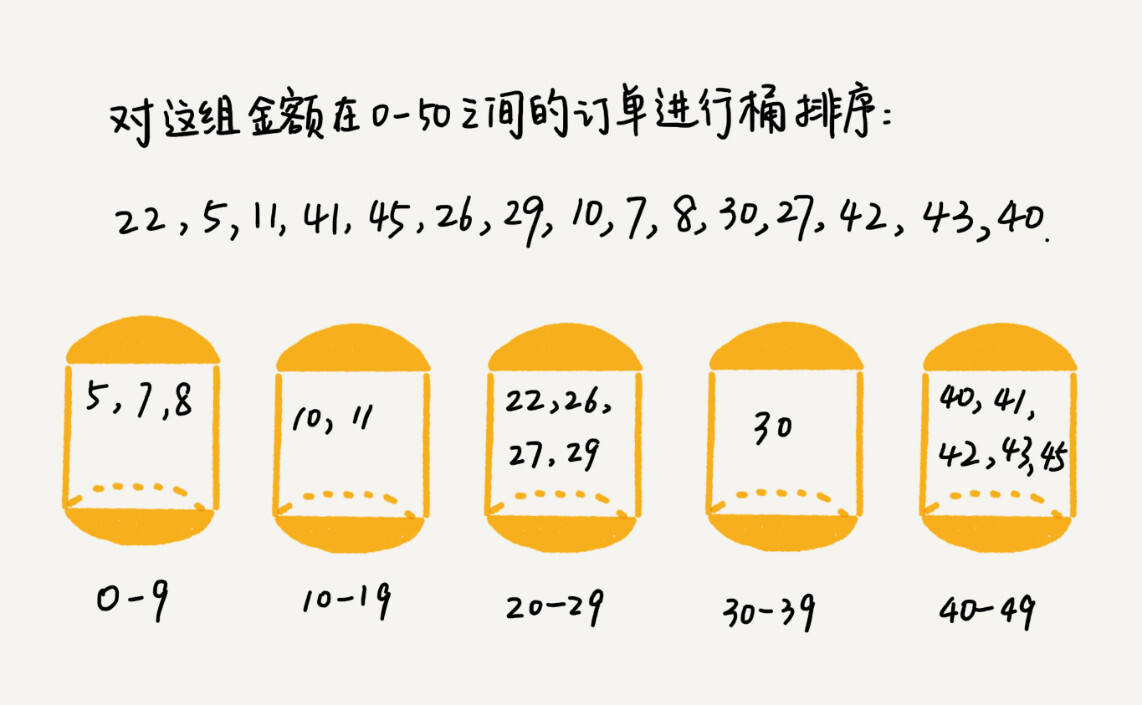#### 计数排序(Counting Sort)

C元素 2 0 2 3 0 1
C下标 0 1 2 3 4 5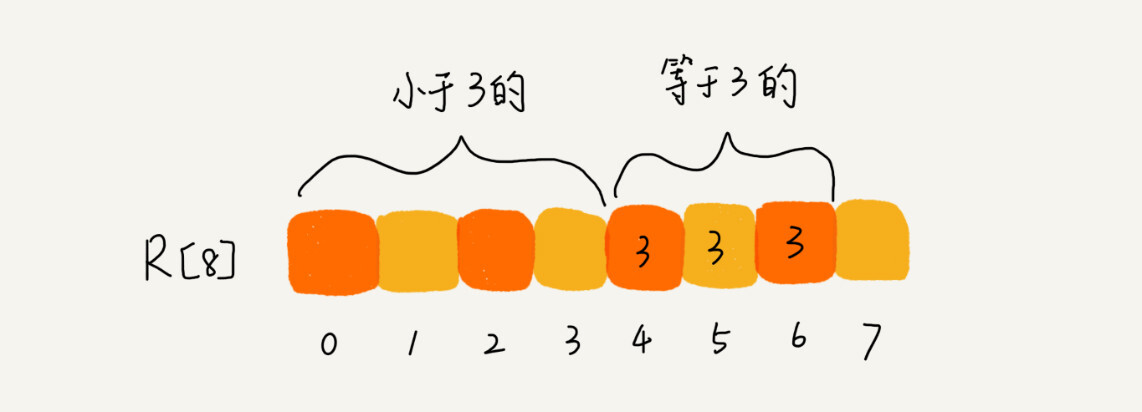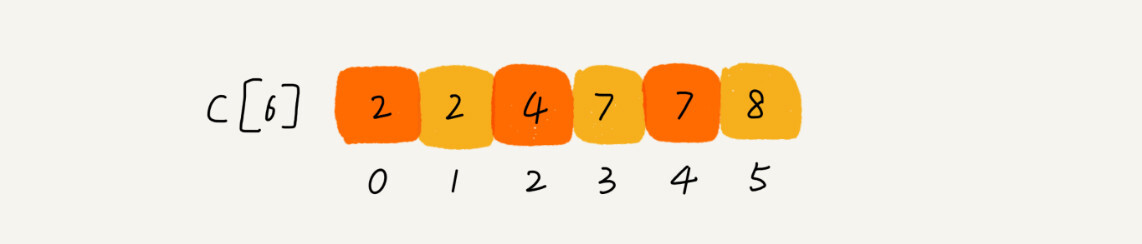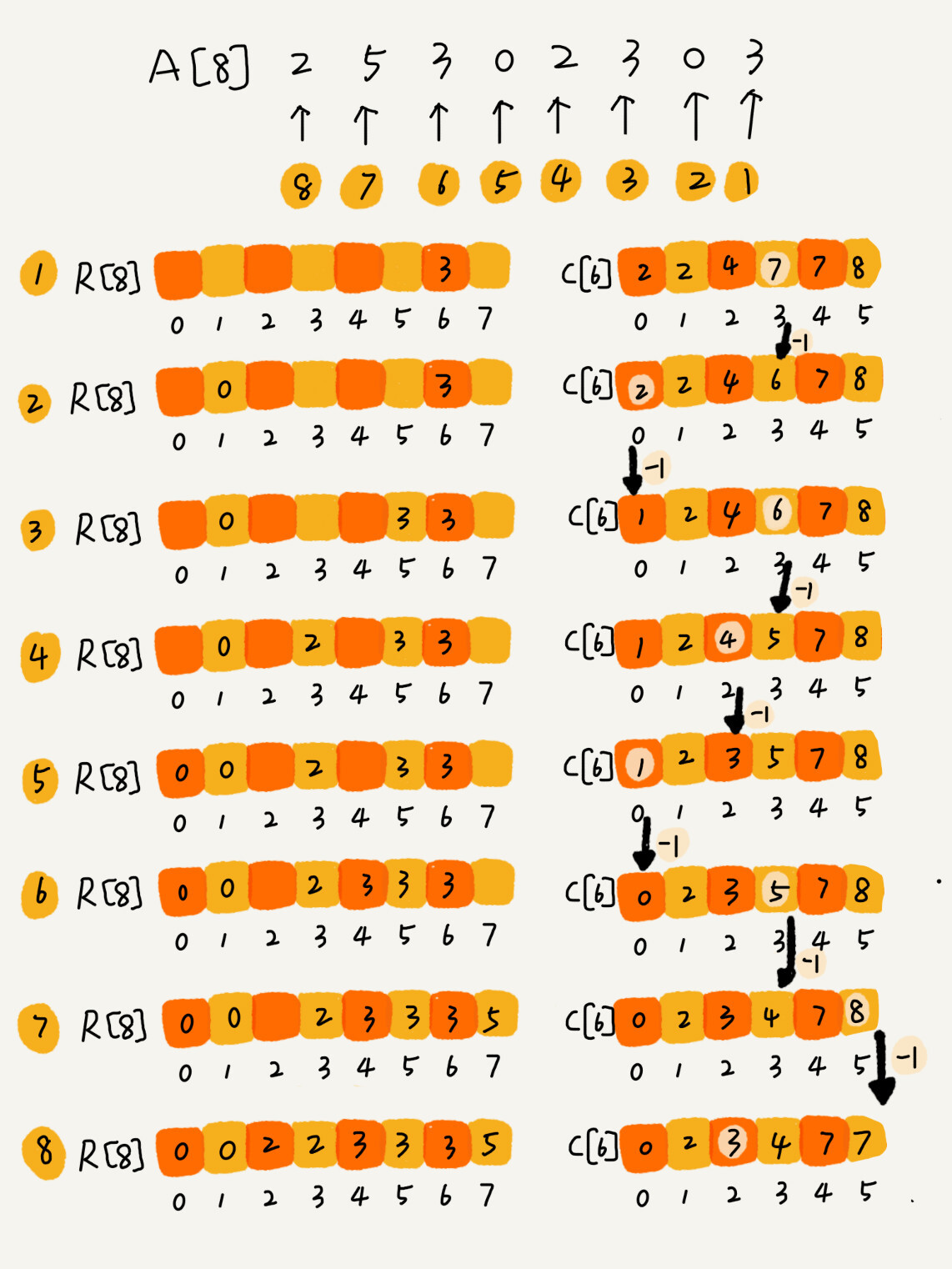``````//计数排序，a是数组，n是数组大小。假设数组中存储的都是非负整数。
public void countingSort(int[] a, int n) {
if (n <= 1) return;

//查找数组中数据的范围
int max = a;
for (int i = 1; i < n; i++) {
if (max < a[i]) {
max = a[i];
}
}

//申请一个计数数组c，下标大小[0, max]
int[] c = new int[max + 1];
//Java语言可以不进行这一步赋值0操作，会默认初始值都为0
for (int i = 0; i <= max; i++) {
c[i] = 0;
}

//计算每个元素的个数，放入数组 c 中
for (int i = 0; i < n; i++) {
c[a[i]]++;
}

//依次累加数组c中的元素
for (int i = 1; i <= max; i++) {
c[i] = c[i-1] + c[i];
}

//临时数组r，存储排序之后的结果
int[] r = new int[n];
//计数排序的关键步骤，比较难理解
for (int i = n - 1; i >= 0; i--) {
int index = c[a[i]] - 1;
r[index] = a[i];
c[a[i]]--;
}

//将结果拷贝给a数组
for (int i = 0; i < n; i++) {
a[i] = r[i];
}
}
``````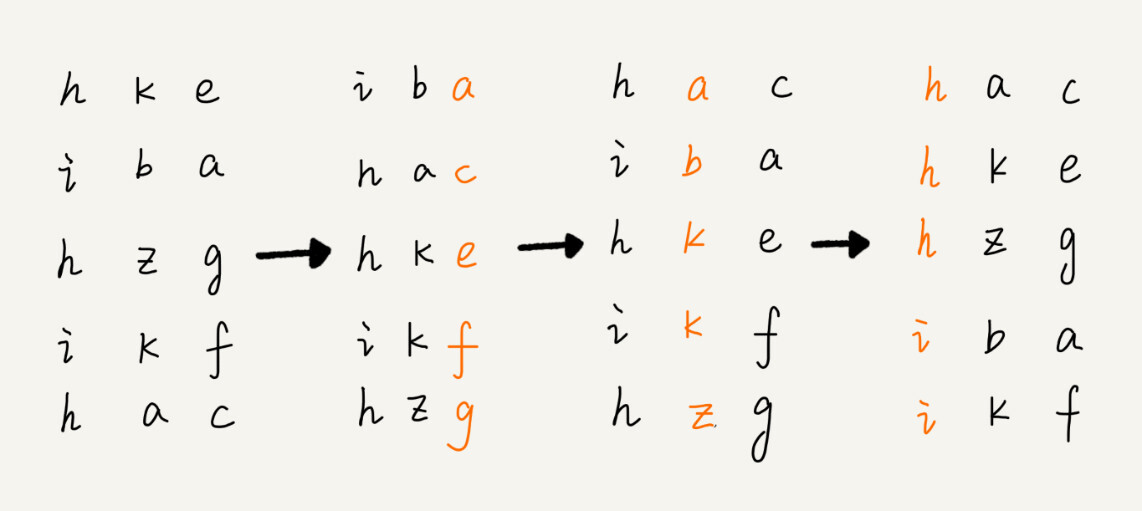#### 思考

1. 这里的桶排序桶内部排序要保证稳定性，所以不能使用快排，得使用归并排序。  2

2. 可以用两个指针a、b：a指针从头开始往后遍历，遇到大写字母就停下，b从后往前遍历，遇到小写字母就停下，交换a、b指针对应的元素；重复上述过程，直到a、b指针相交。对于小写字母放在前面，数字放中间，大写字母放后面，可以先将数据分为小写字母和非小写字母两大类，进行如上交换后再在非小写字母区间内分为数字和大写字母做同样处理。